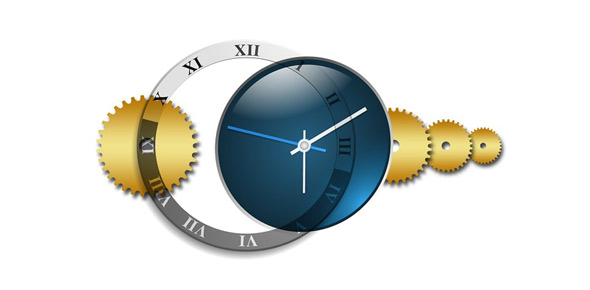# Trivia Year, Month, Week, Day, Hour, Minute And Second

16 Questions | Attempts: 427
ShareSettingsTime is one of the most precious and scarce resources available to man today. Do you adore time and take it seriously? This quiz will help you know more about it all.

• 1.
A common year has how many days?
• A.

360 days

• B.

365 days

• C.

366 days

• D.

364 days

• 2.
How many weeks are in a year?
• A.

48

• B.

51

• C.

53

• D.

52

• 3.
Which years are leap years?
• A.

2007

• B.

2008

• C.

2010

• D.

2011

• E.

2012

• 4.
A leap year has how many days?
• A.

364

• B.

365

• C.

366

• D.

367

• 5.
In which month is in a leap year ONE day added?
• A.

January

• B.

June

• C.

August

• D.

February

• E.

April

• 6.
One day has how many hours?
• A.

20

• B.

23

• C.

26

• D.

24

• E.

25

• 7.
One week has how many hours?
• A.

175

• B.

150

• C.

168

• D.

165

• 8.
How many seconds are in one hour?
• A.

360

• B.

3000

• C.

3600

• D.

2400

• 9.
How much is 1 hour 40 min + 30 min
• A.

1 hour 70 min

• B.

2 hours 10 min

• C.

2 hours 20 min

• 10.
It is now  9:25amWhat will the time be in 3 hours and 55 minutes?
• A.

12:80am

• B.

1:20pm

• C.

2:20pm

• 11.
How many minutes are in a quarter of an hour?
• A.

25 min

• B.

30 min

• C.

15 min

• D.

40 min

• 12.
What is the time in the 24 hour format at 3:25pm
• A.

03:25

• B.

16:25

• C.

15:25

• 13.
What is the time in the 12 hour format at 18:55
• A.

8:55am

• B.

6:55pm

• C.

5:55pm

• 14.
In which century are we now?
• A.

19th century

• B.

21st century

• C.

20th century

• 15.
4,722 seconds are how many hours, minutes and seconds?
• A.

2 hours, 8 minutes and 22 seconds

• B.

1 hour, 18 minutes and 42 seconds

• C.

57 minutes and 22 seconds

• 16.
3,658 minutes are how many days, hours and minutes?
• A.

3 days and 58 minutes

• B.

3 days, 6 hours and 58 minutes

• C.

2 days, 12 hours and 58 minutes

## Related TopicsBack to top
×

Wait!
Here's an interesting quiz for you.